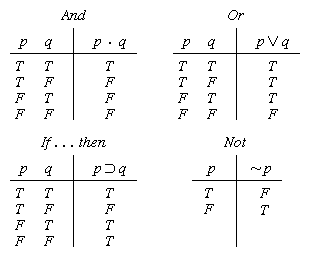Truth-value | logic | Britannica

# Truth-value

logic

Truth-value, in logic, truth (T or 1) or falsity (F or 0) of a given proposition or statement. Logical connectives, such as disjunction (symbolized ∨, for “or”) and negation (symbolized ∼), can be thought of as truth-functions, because the truth-value of a compound proposition is a function of, or a quantity dependent upon, the truth-values of its component parts.

The truth-value of a compound statement can readily be tested by means of a chart known as a truth table. Each row of the table represents a possible combination of truth-values for the component propositions of the compound, and the number of rows is determined by the number of possible combinations. For example, if the compound contains just two component propositions, there will be four possibilities and thus four rows to the table. The logical properties of the common connectives may be displayed by truth tables as follows:in which · signifies “and” and ⊃ signifies “if . . . then.” (In the “or” table, for example, the second line reads, “If p is true and q is false, then p q is true.”) Truth tables of much greater complexity, those with a number of truth-functions, can be constructed by means of a computer. Abstract systems of logic have been constructed that employ three truth-values (e.g., true, false, and indeterminate) or even many, as in fuzzy logic, in which propositions have values between 0 and 1.